﻿ Derivation of Geometric Mean Beam Length Relations
 A: Derivation of Geometric Mean Beam Length Relations   The Geometric Mean Beam Lengths depend on both geometry and wavelength through the definitions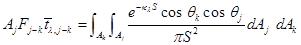(A-1)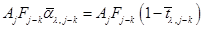(A-2)   The double integral in (A-1) must be evaluated for various orientations of surfaces Aj and Ak; the result will depend on kλ.  Derivations for some specific geometries are now considered.   A.1 Hemisphere to Differential Area at Center of its Base Let Aj be the surface of a hemisphere of radius R, and dAk be a differential area at the center of the base (Fig. A-1). Then (A-1) becomes, since S=R and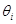= 0,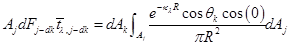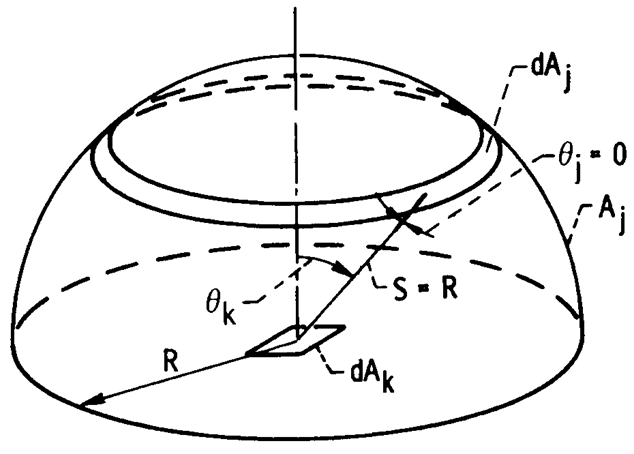FIGURE A-1 Hemisphere filled with isothermal medium.   The convenient dAj is a ring element dAj = 2πR2 sin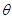k dk, and the factors involving R can be taken out of the integral. This gives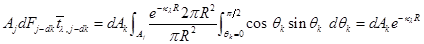With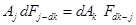and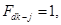, this reduces to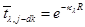(A-3)   This especially simple relation is used later in the concept of mean beam length where radiation from an actual volume of a medium is replaced by that from an equivalent hemisphere.